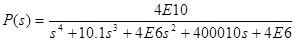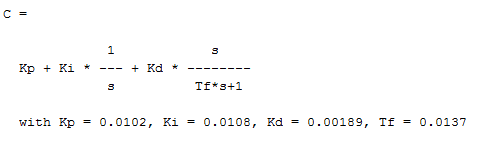# Making a plant stable with a compensator based on given requ

• shuh
In summary, the student is trying to design a compensator for a transfer function of a plant. The compensator must have a control bandwidth of 100 rad/s, a minimum phase margin of 30 deg, and an overshoot of 20% at 2000 rad/s. The compensator must settle in 0.75 s, and the steady-state error to the unit step reference must be less than 1%. The compensator must have a gain margin of 22 dB.f

## Homework Statement## The Attempt at a Solution

For the above modeled transfer function of the plant, I'm trying to design a compensator that satisfies some requirements.

a. The maximum control bandwidth (0 dB crossover frequency) is 100 rad/s.

b. The minimum phase margin at crossover is 30 deg.

c. The loop transmission magnitude at 2000 rad/s must be less than -6 dB.

d. The step response overshoot of the closed loop system must be less than 20%.

e. The 2% settling time must be less than 0.75 s.

f. The steady-state error to the unit step reference is less than 1%.

Open loop poles reside in -0.05 +/- i and - 5 +/- 2000i, so there is a lot of potential for instability for some variable gain K. This means that I would need a two zeros for the poles to sink in.

Designing a compensator that makes this system stable is easy, I can throw in two zeros in the LHP such as s^2 + 2 s + 8. What I'm not too sure on is the method of satisfying the requirements. Normally, I'd use a PID controller to tune the settling time, step response etc. But in this system, a PID controller cannot be applied, because the addition of pole would make this system unstable. (Edit: Well I guess I could use PID, as long as I define the bounds for gain K, but this seems like a crummy solution as the range of K would be really small)

What are some alternative methods of achieving the requirements while making the system stable?

: http://i.stack.imgur.com/SoB2j.png

Designing a compensator that makes this system stable is easy, I can throw in two zeros in the LHP such as s^2 + 2 s + 8.
The trouble with compensators that have more zeros than poles is that they're noncausal systems, i.e. they're unrealizable.

Have you had a look at this system in the frequency domain? You have two awful resonance peaks, but in between them is an area of almost constant -180 deg phase angle, where you could add a lead compensator and adjust the gain to get crossover on its frequency of maximum phase lead.

This assignment looks designed to show you a compromise. You need the phase lead at a high enough frequency to get you enough closed-loop bandwidth, and you need enough low-frequency gain to reduce the steady-state error. Both of these requirements "lifts up" the high-frequency resonance peak and thus lowers your gain margin. There's a compromise that fits your requirements, however.

•donpacino
The trouble with compensators that have more zeros than poles is that they're noncausal systems, i.e. they're unrealizable.

Have you had a look at this system in the frequency domain? You have two awful resonance peaks, but in between them is an area of almost constant -180 deg phase angle, where you could add a lead compensator and adjust the gain to get crossover on its frequency of maximum phase lead.

This assignment looks designed to show you a compromise. You need the phase lead at a high enough frequency to get you enough closed-loop bandwidth, and you need enough low-frequency gain to reduce the steady-state error. Both of these requirements "lifts up" the high-frequency resonance peak and thus lowers your gain margin. There's a compromise that fits your requirements, however.

Looking at the root locus plot for the plant, it seems like the gain margin is really small to begin with. If I'm going to add a phase lead at high frequency at the cost of gain margin, to me it seems like might as well as use a PID controller to easily satisfy the requirement. So am I right to assume that there truly is no way of satisfying all of the requirements above while having a reasonable gain margin?

So I designed a PID controller that looks like the following:which I think does satisfy the requirements looking at the step response and the bode plot, of course at the cost of nearly no gain margin. Would this be a good solution?

So am I right to assume that there truly is no way of satisfying all of the requirements above while having a reasonable gain margin?
I see more than 22 dB margin for both the lower and upper bound on the gain for the cascade of your controller and plant. That's very decent.

The lower bound is something you introduce by adding a pure integrator (pole at zero, component of PID), which isn't present with the lead compensator.

For high-order systems, I like using the frequency response for design, since, in my experience, it's rare in practice to have a pole pair be truly dominant. The system response is then due to the compound effects of a whole bunch of stuff, but the rules for loop shaping stay the same. Maybe you feel the same about root-locus design.